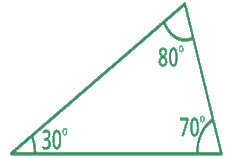1
visibility

What kind of triangle is this?• Acute Triangle

• Obtuse Triangle

• Right Triangle

The correct answer is "Acute Triangle".

Solution:

Consider the triangle below.Notice that all the three angles of this triangle are less than 90°.

So, it is an Acute Triangle.